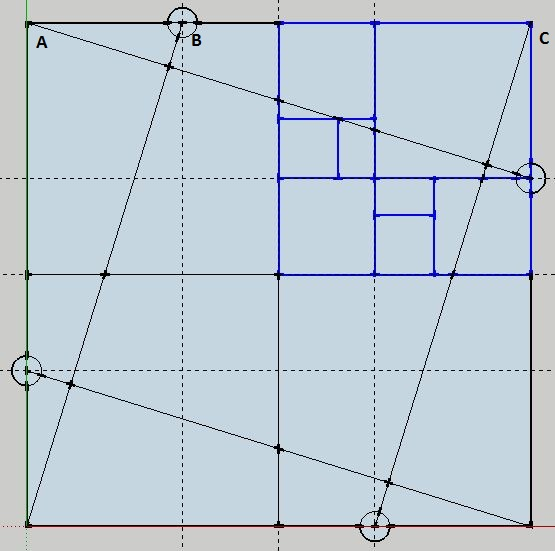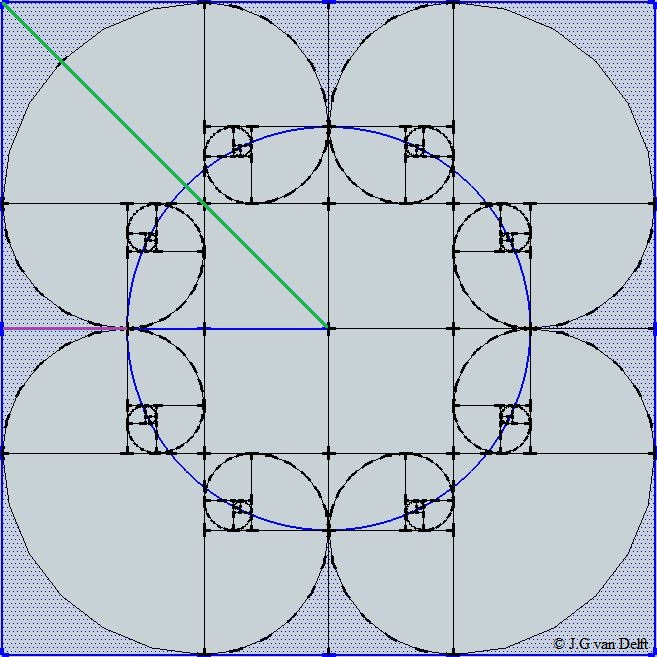Home | Study Links | Poems |

## 8 Golden Ratio SpiralsThis geometric configuration starts with lines ab .5 + be √1.25 = ae ϕ
Measurements for ae = ϕ, ge = .5, ag = √(ϕ + 1.25)Golden Rectangles Construction:
Draw guide lines A and B parallel to the square’s external margins, and connect them to the small circled junction points. In this 2-dimensional diagram there are 8 Golden ratio spirals.The point to which all golden rectangles converge is sometimes called the “Eye of God”. Here 8 golden rectangles and their converging points form a circle, and a radius (R) which is equal to the sides of length of the square (L).

D / R = (√5 + 3) is one example of interesting ratios that can be found within this diagram.
Representing one face of a three-dimensional cube, there are 48 golden rectangles in total.

Measurements and ratios that occur in this geometric drawing, show that there is one arithmetical number
that is prominent, and that number is 5. In Biblical scripture the number 5 symbolizes “Grace”.